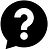top of page

JC Mathematics###### ​

H2 Mathematics is designed to train students to be analytic problem solvers with the aim of preparing them for a range of university courses across various disciplines. The syllabus trains students to connect ideas within mathematics and apply mathematics to real world context related to these disciplines. In doing so, students can experience and appreciate the nature and beauty of mathematics and its value in life.

In H2 Mathematics, students will be exposed to two branches: Pure Mathematics and Statistics. Pure Mathematics heavily relies on our understanding of Additional Mathematics. While Statistics relies on only (Elementary) Mathematics. The learning curve for the transition from Secondary Mathematics (regardless of whether a student is in the O-Level and IP streams) to both these branches in H2 Mathematics is steep.

Lesson Structure

There are Six (6) H2 Mathematics modules (or chapters) in total. Every module entails three types of lessons. We start with a few lessons on Lecture & Practice, before proceeding to A-Level Revision and finally, Preliminary Exam Revision lessons.

a)   Lecture & Practice

• Concept Development

• Practice (with step-by-step solutions) - Basic Practice, Guided Practice & Self-Practice​

​b)   A-Level Revision: Carefully curated A-Level Questions with step-by-step solutions.

c)   Preliminary Exam Revision: Carefully curated Prelim Questions with step-by-step solutions.

Our lessons provide personalized learning and structured guidance to help students cope with this transition. The lessons are conducted as modules where it will be repeated at certain periods throughout the academic year to accommodate the schedules of as many Junior College students as possible.

Learning Methodology

Each module facilitates Personalized Learning in two ways:

• Three-tiered Practice in Lecture & Practice to cater to different levels of competency in a chapter;

• Three-tiered Learning that starts with the 'Foundation' level in Lecture & Practice, then 'Intermediate' level in A-Level Revision and finally, the 'Difficult' level for the JC2 Preliminary Examinations.

Each module provides Structured Guidance in three ways:

• In school lectures, students are taught concepts with little time to digest each before proceeding to the next concept. In our Lecture & Practice, we go through an Example (and concept) in Concept Development and proceed to do a corresponding Basic Practice question for an application of the concept taught. We do Examples and Basic Practice questions in tandem;

• The struggle with most students with this subject is starting on a given practice question. Besides a three-tiered practice, the Self-Practice questions (which are assigned as additional practice) have hints attached to them so that students can start the question;

• For A-Level and Prelim questions, we link each part of the question to concepts taught in the previous weeks in Lecture & Practice. This crystallizes the thought process required for exam questions of that chapter.

Personalized Learning and Structured Guidance together hopes for a coherent learning experience for the student in each module.

Lesson Objectives

Our Mathematics modular lessons aim to develop the following in JC students:

• Build a strong foundation by mastering the key fundamental concepts in H2 Mathematics;

• Sharpen the analytical skills and techniques that are required to tackle challenging questions in JC exams & the A-Level Mathematics exam; and

• Cultivate interest in Mathematics so that learning becomes practical and effective.

To accommodate the schedules of as many JC students as possible, our H2 Mathematics Modules will be conducted and repeated at certain periods throughout the academic year.  There are SIX (6) H2 Mathematics modules in total. To learn more, please click the respective module icons below.#### 6 H2 Mathematics ModulesSequence & SeriesDifferentiationIntegrationVectorsProbabilityDiscrete Random

VariableIntroduction to JC Mathematics (FOC)Excelling in A-Level H2 Mathematics (FOC)H2 Mathematics

### For JC1 & JC2 Students

•